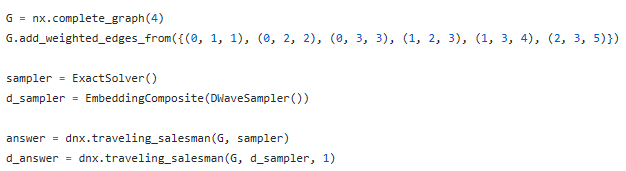# Traveling Salesman Problem (TSP)

The Jupyter Notebook for the TSP has a 7 city and a 48 city example with executable code.  Both seem to use qbsolv in the classical mode.

1.  Is it correct to conclude that no quantum effects are simulated?

2.  What modifications are needed so that the 48 city example will execute qbsolv in a hybrid manner (classical-QPU combination)?

3.  Suppose we want to change the 48 city example to run another TSP.  I recognize that N = the number of cities and the distance matrix need to be changed.  What else in the code needs to be modified?

Thanks,  Richard H. Warren

1. As the Jupyter Notebook mentions, the QPU is not being used in this example.

2. You can use the following code to enable the QPU with qbsolv:
from dwave_qbsolv import QBSolv
from dwave.system.samplers import DWaveSampler
from dwave.system.composites import EmbeddingComposite

sampler = EmbeddingComposite(DWaveSampler())
response = QBSolv().sample_qubo(Q, solver=sampler, solver_limit=subqubo_size)
https://docs.ocean.dwavesys.com/projects/qbsolv/en/latest/intro.html

3. What exactly would you like to change? Are you looking to change the total number of cities?
The names and locations of the cities should be updated (the ones listed in TSP_48_capitals.txt).
The distances between cities also need to be updated with new distances (TSP_48_state_capitals.txt).
Number of cities in our problem N needs to be updated to match the number of cities listed in the above two files.
There are two sections in the Jupyter Notebook that add constraints to only allow one value of 1 (and the rest 0) in each row and column of the matrix.
These two sections rely on the value N, so it is important to update that value.

• Hi, I am very interested in the TSP, and I would like to know how to solve this problem using Quantum Annealer. I have searched for a long time about the example of TSP with 7 cities and 48 cities you mentioned above. Unfortunately, I still haven’t found it until now. I would be very grateful if you could provide its URL or original codes.

I have tested the function of dnx.traveling_salesman() with different methods, ExactSolver and DWaveSampler. But the results were completely different. Apparently, the result solved by QPU was wrong. I got unpredictable results - sometimes long list - sometimes list of length one. I don’t why. So if you have such a problem, please tell me how you solved it.

Thanks very much.

Code:• I am just redirecting this post to a similar post with more information:
https://support.dwavesys.com/hc/en-us/community/posts/360028972434